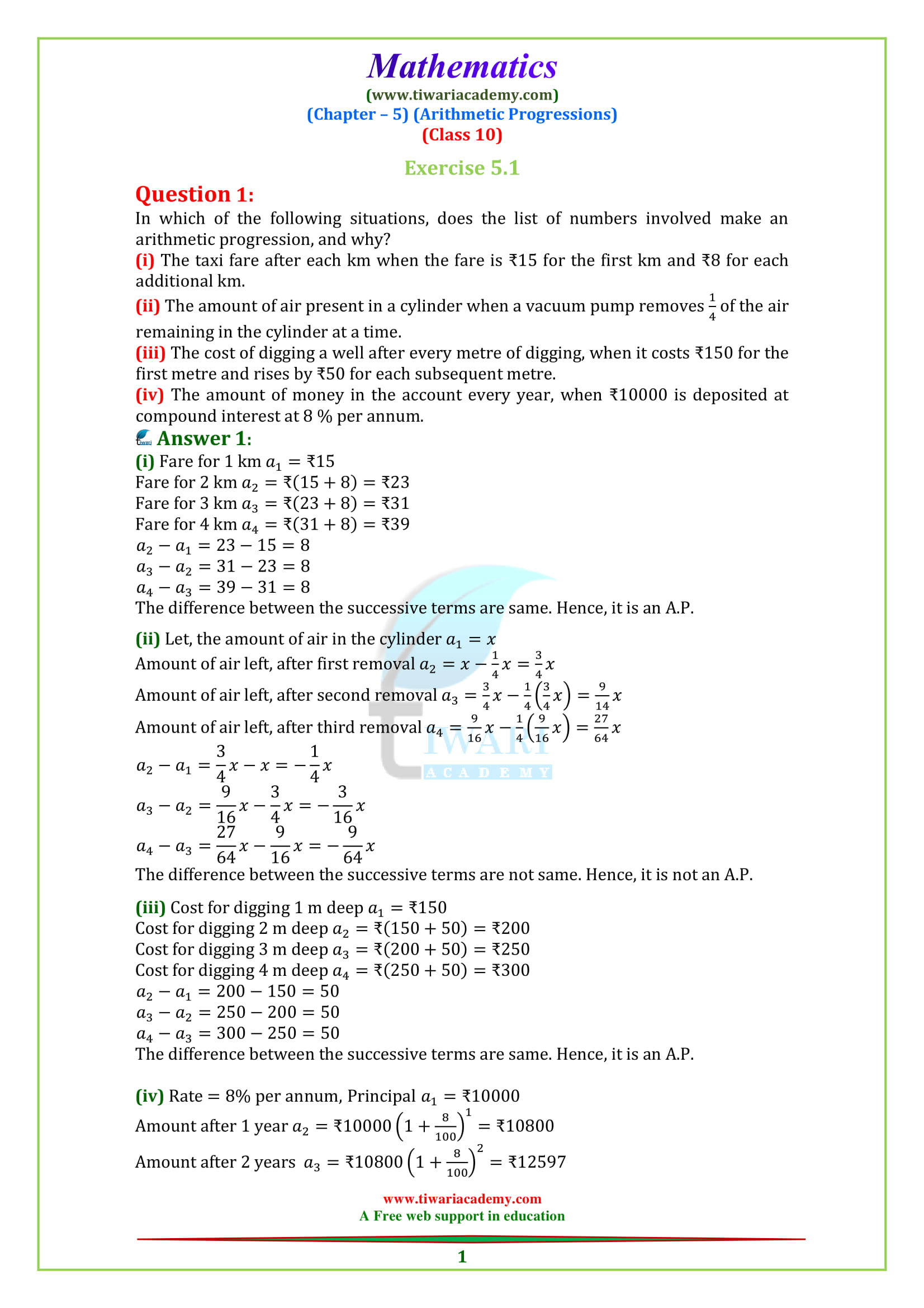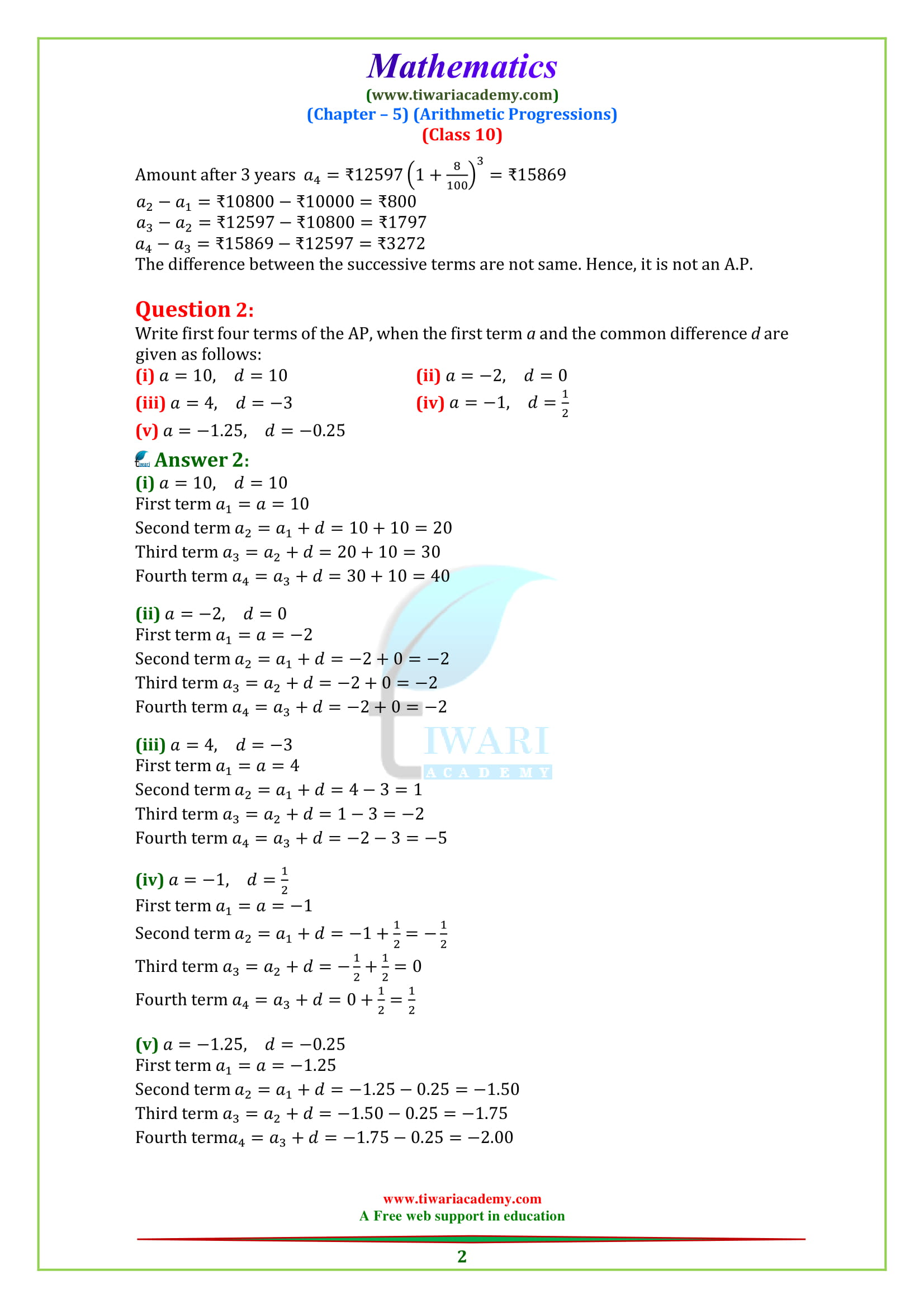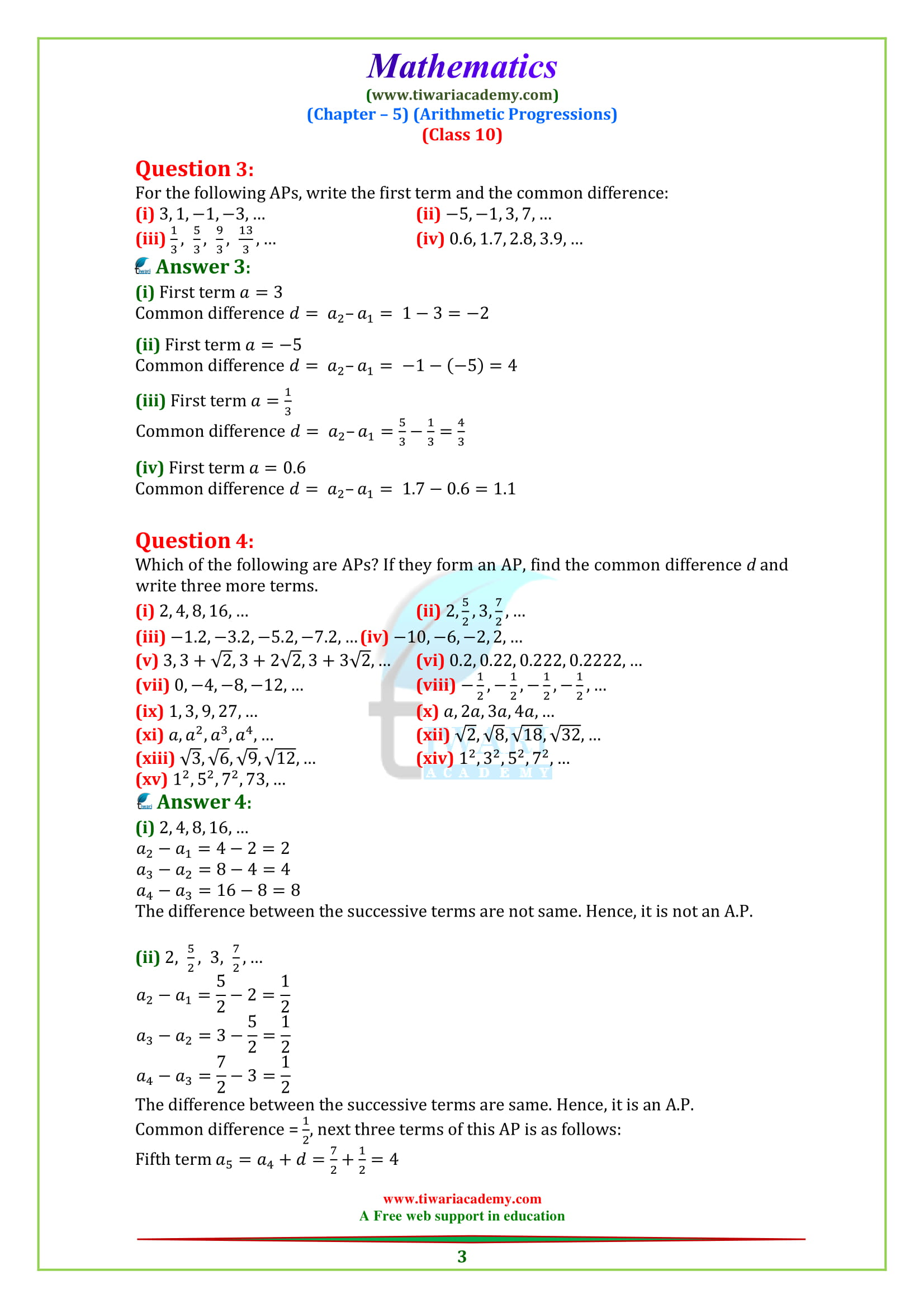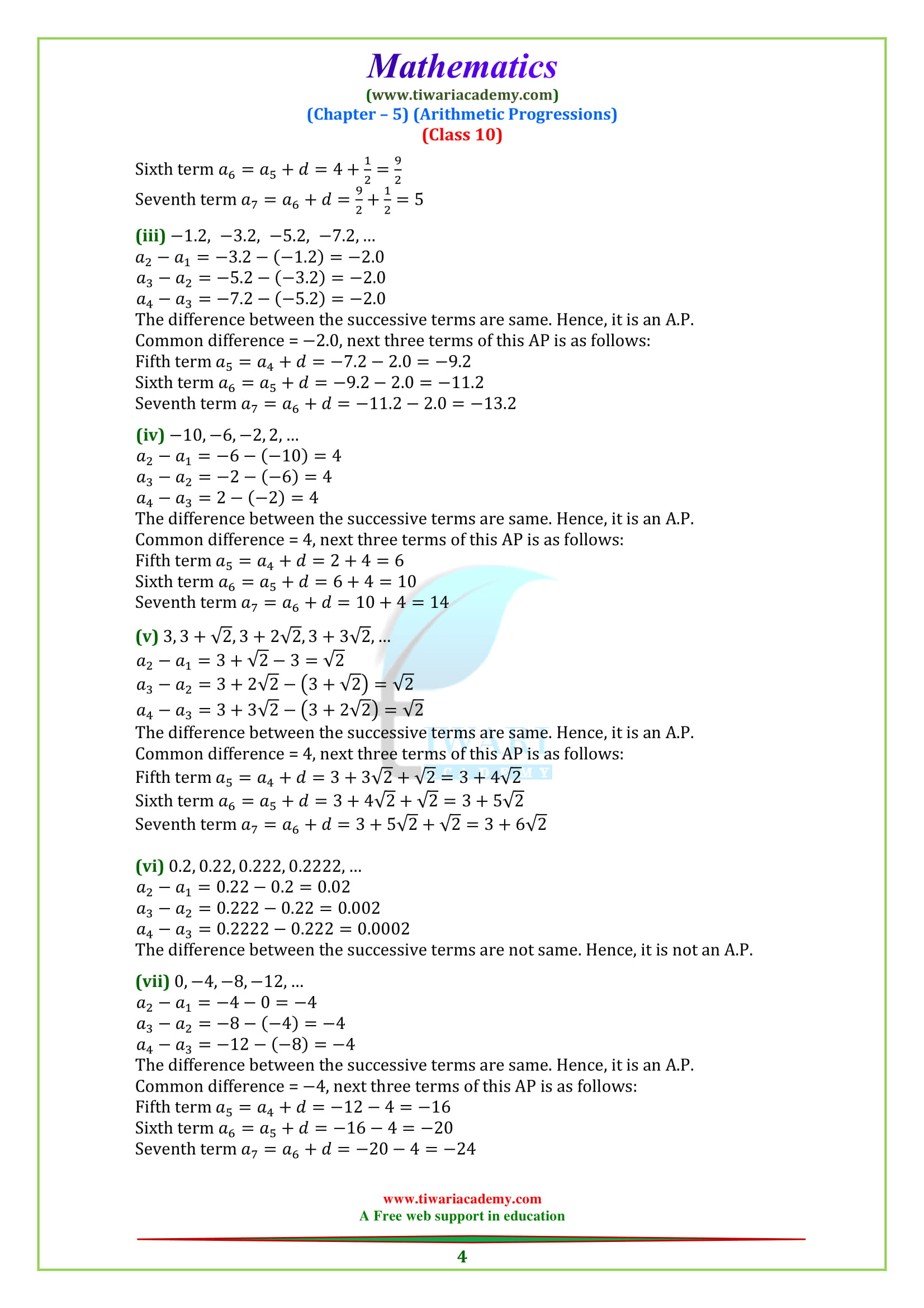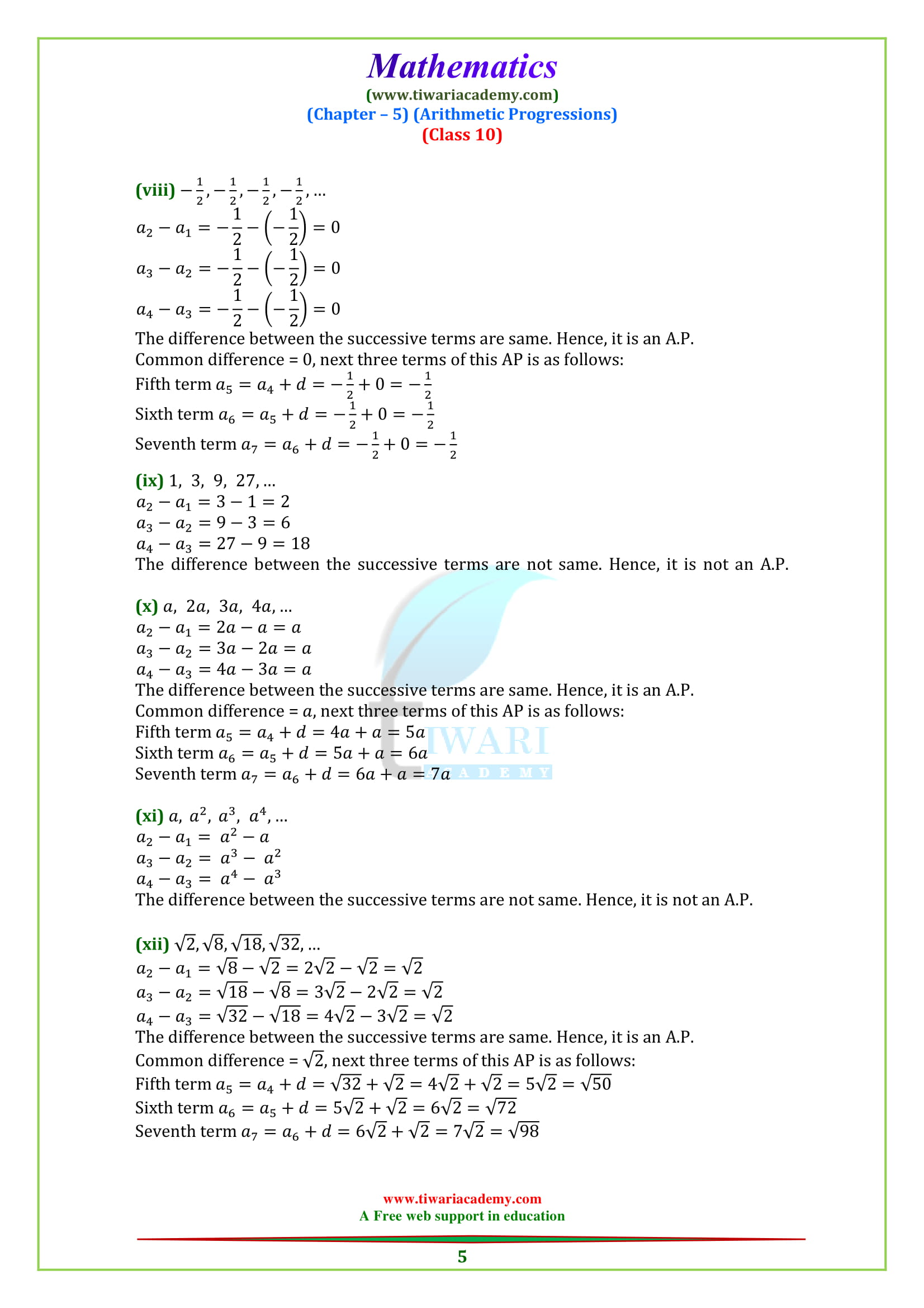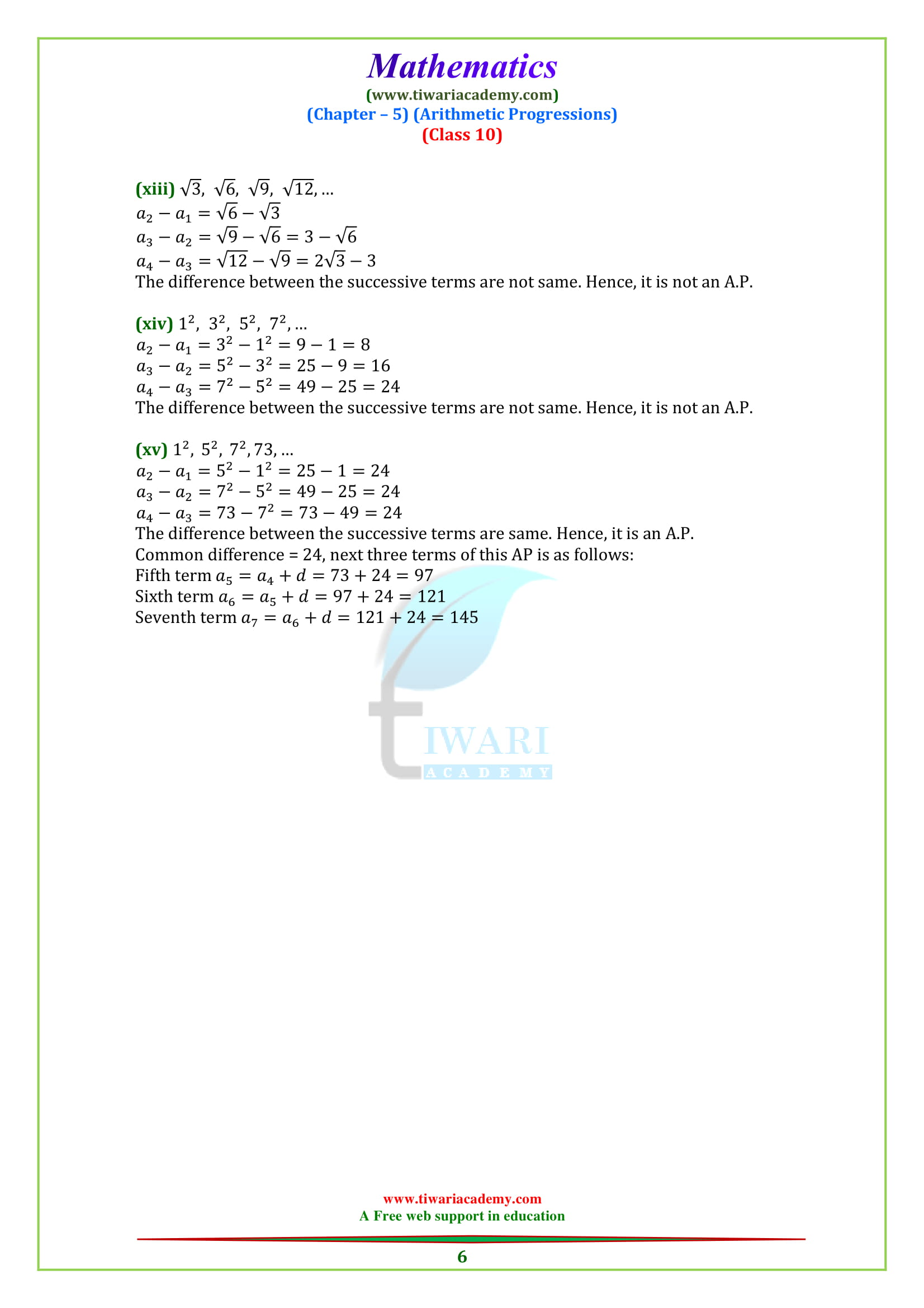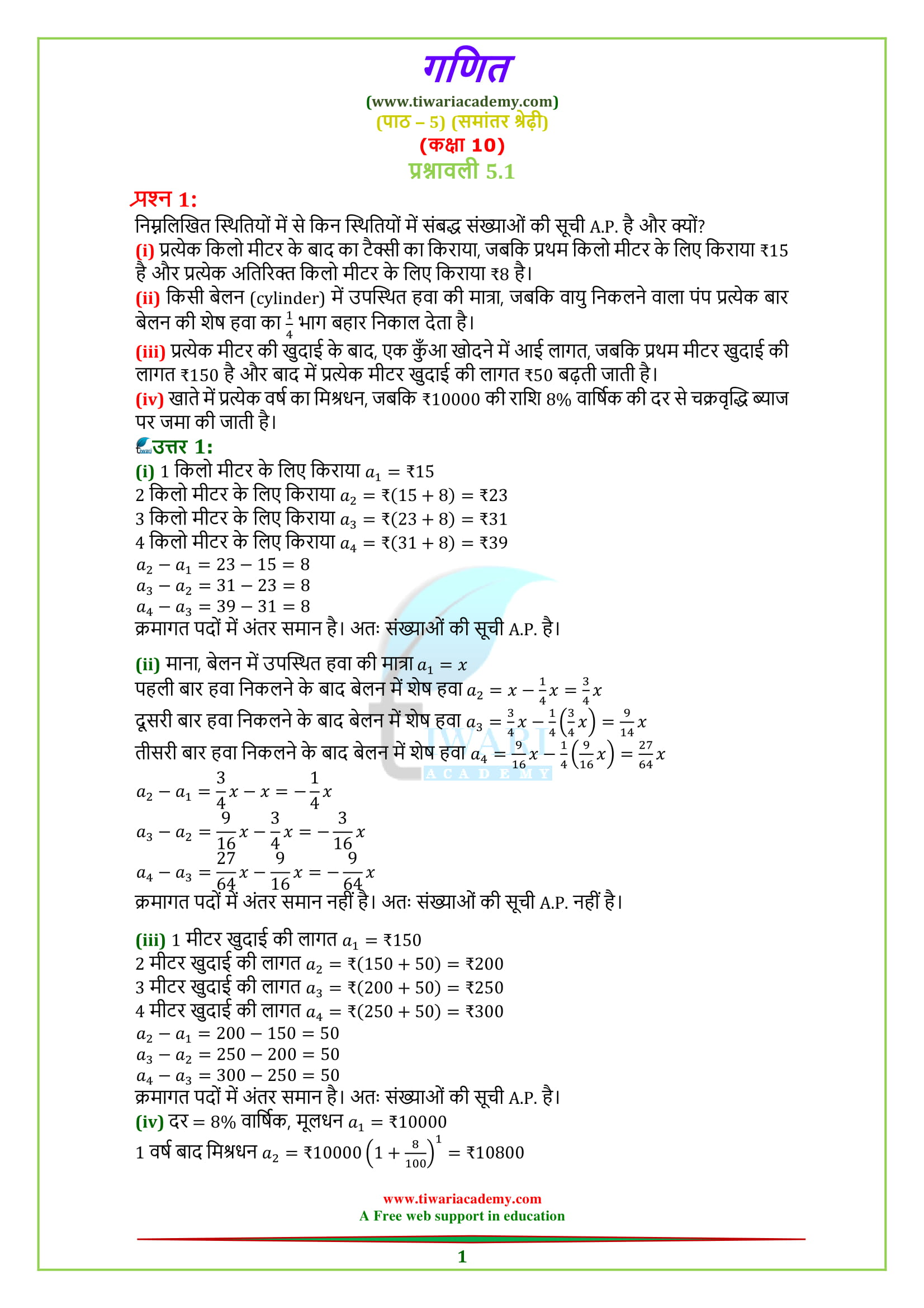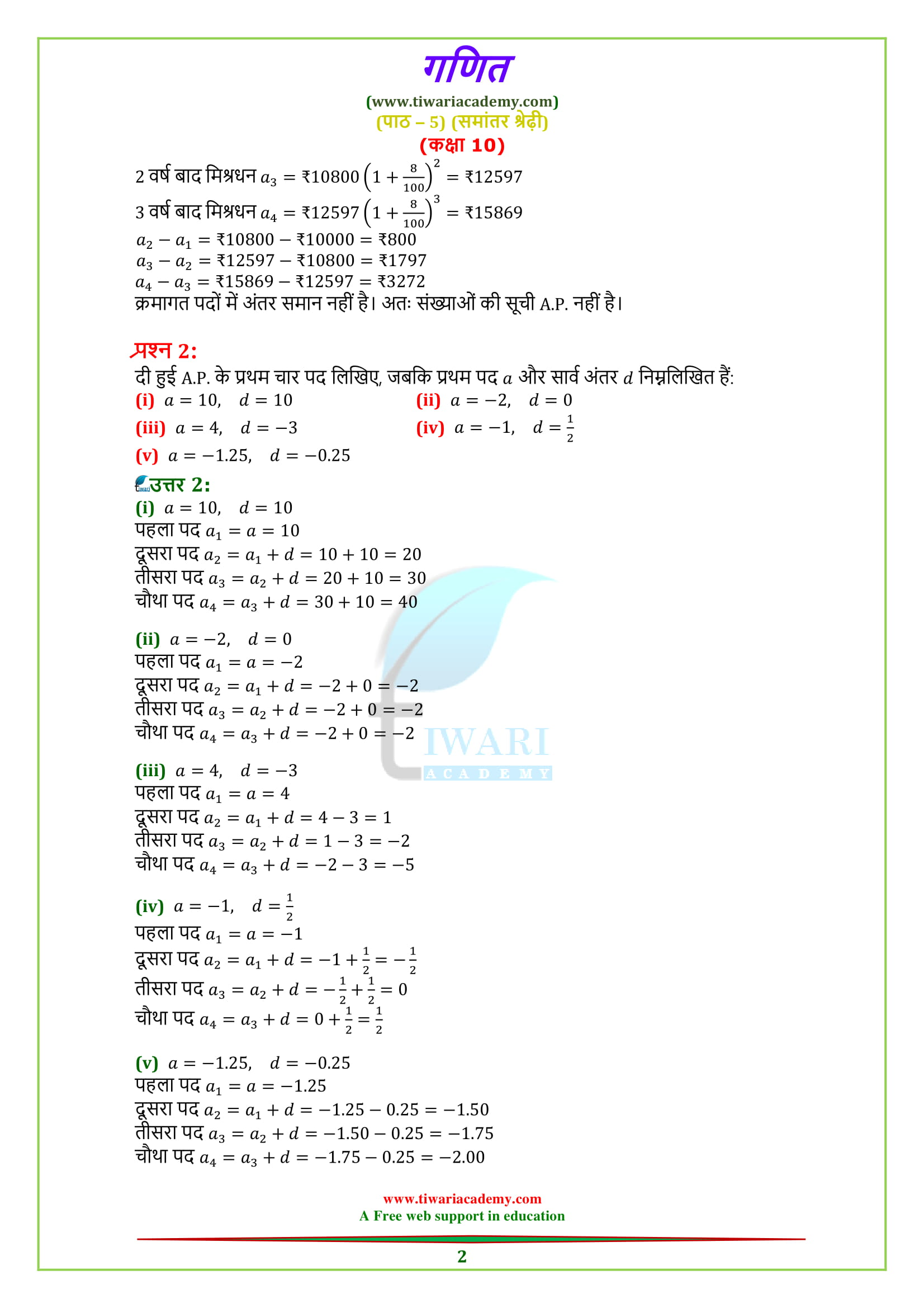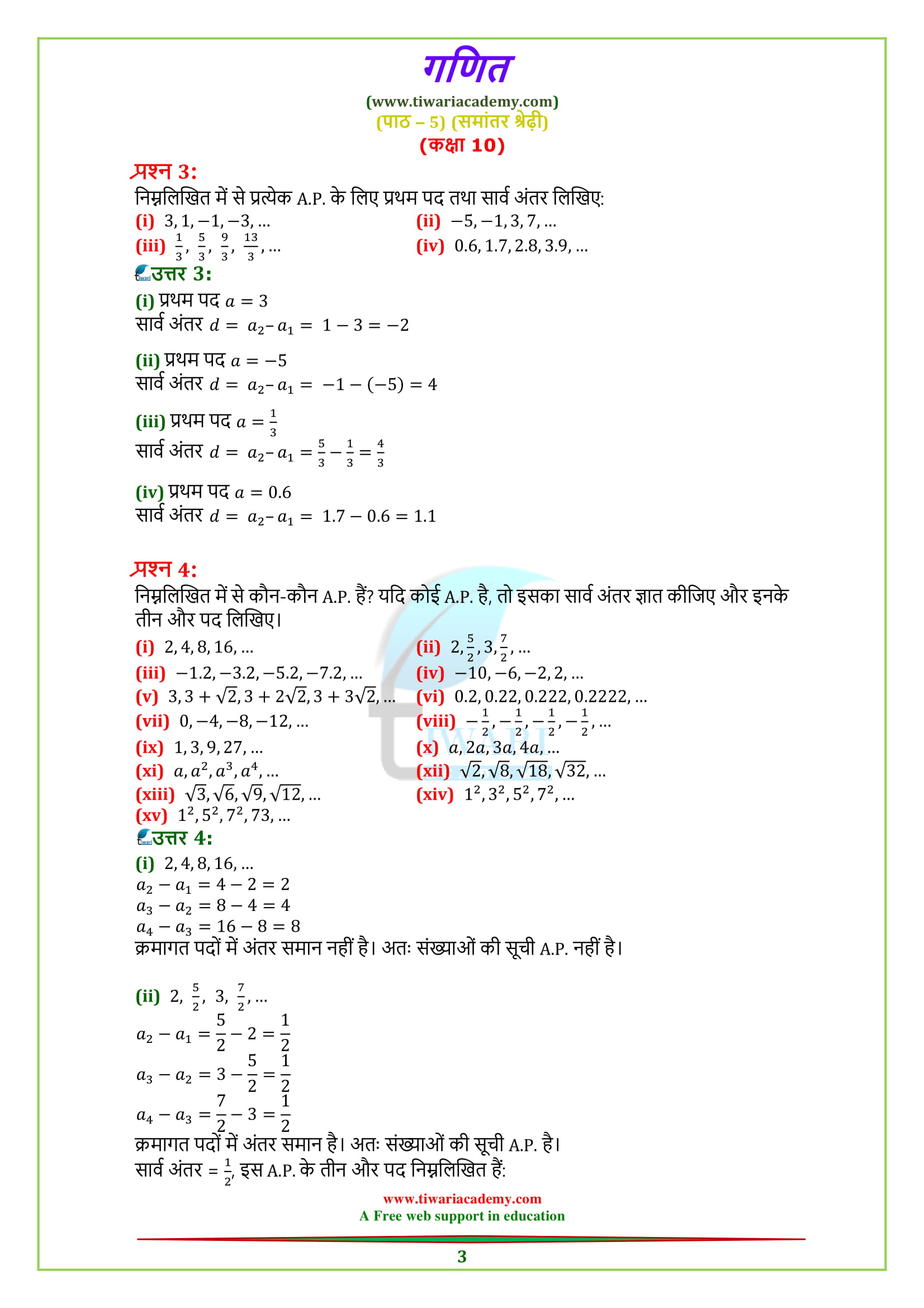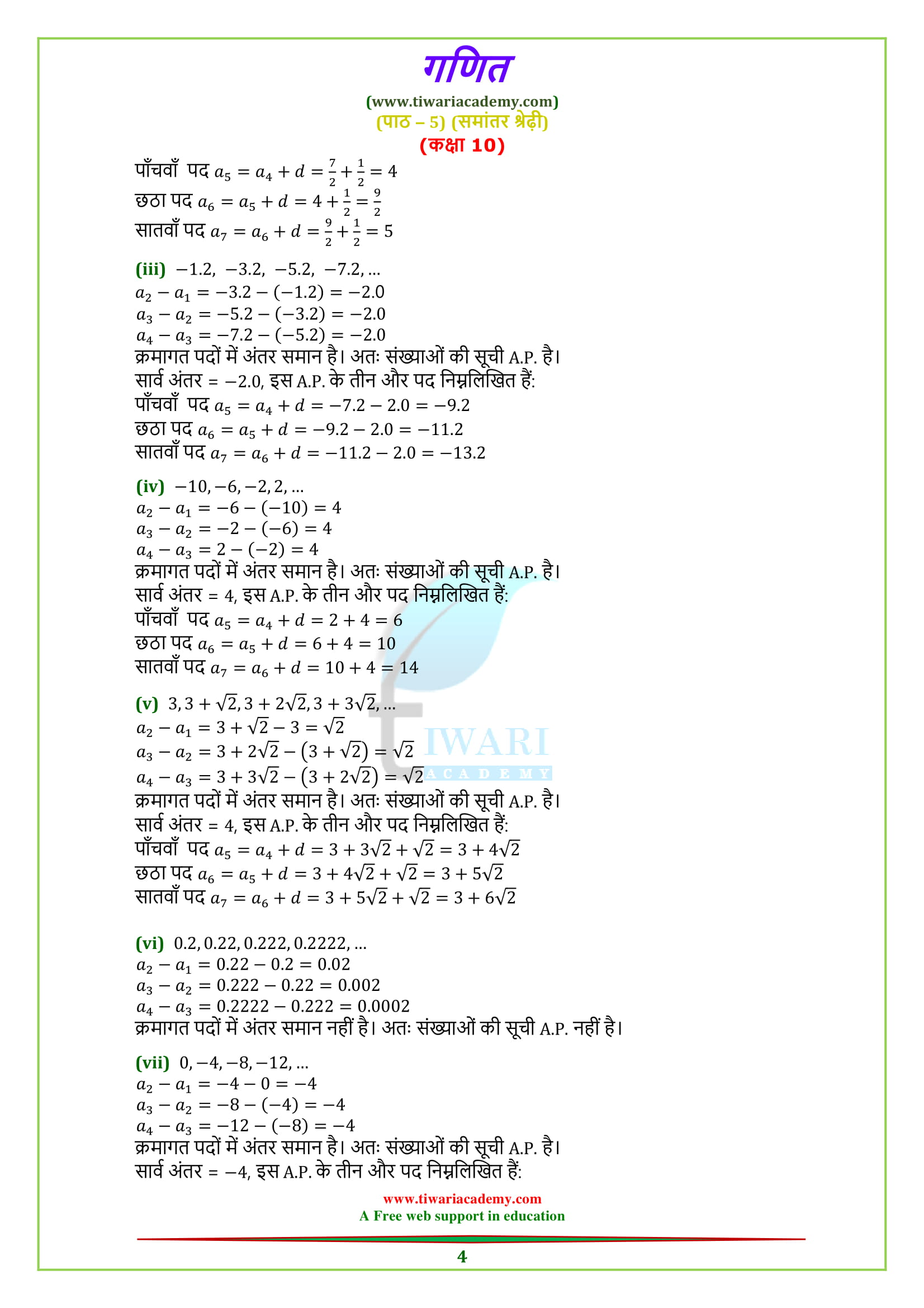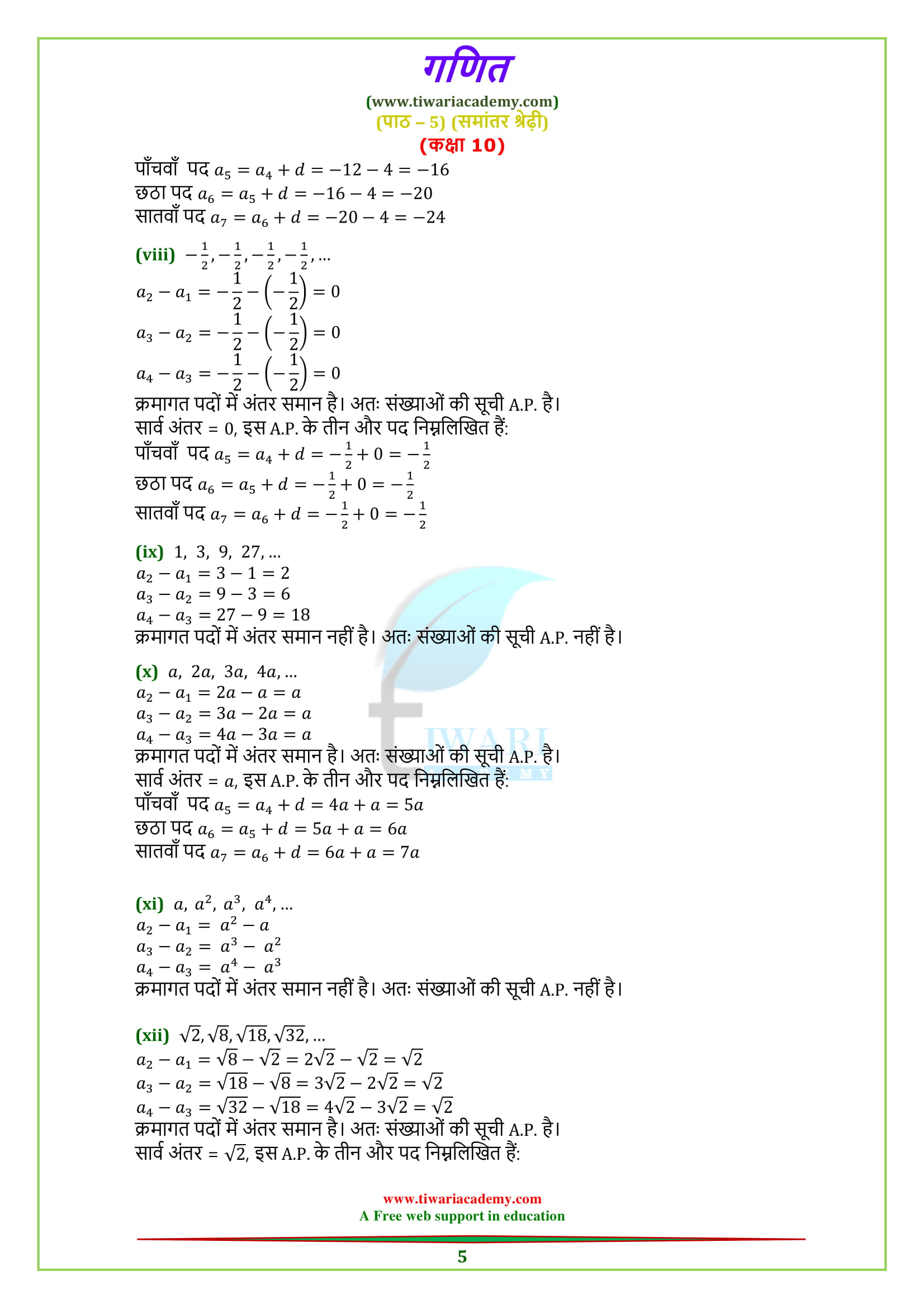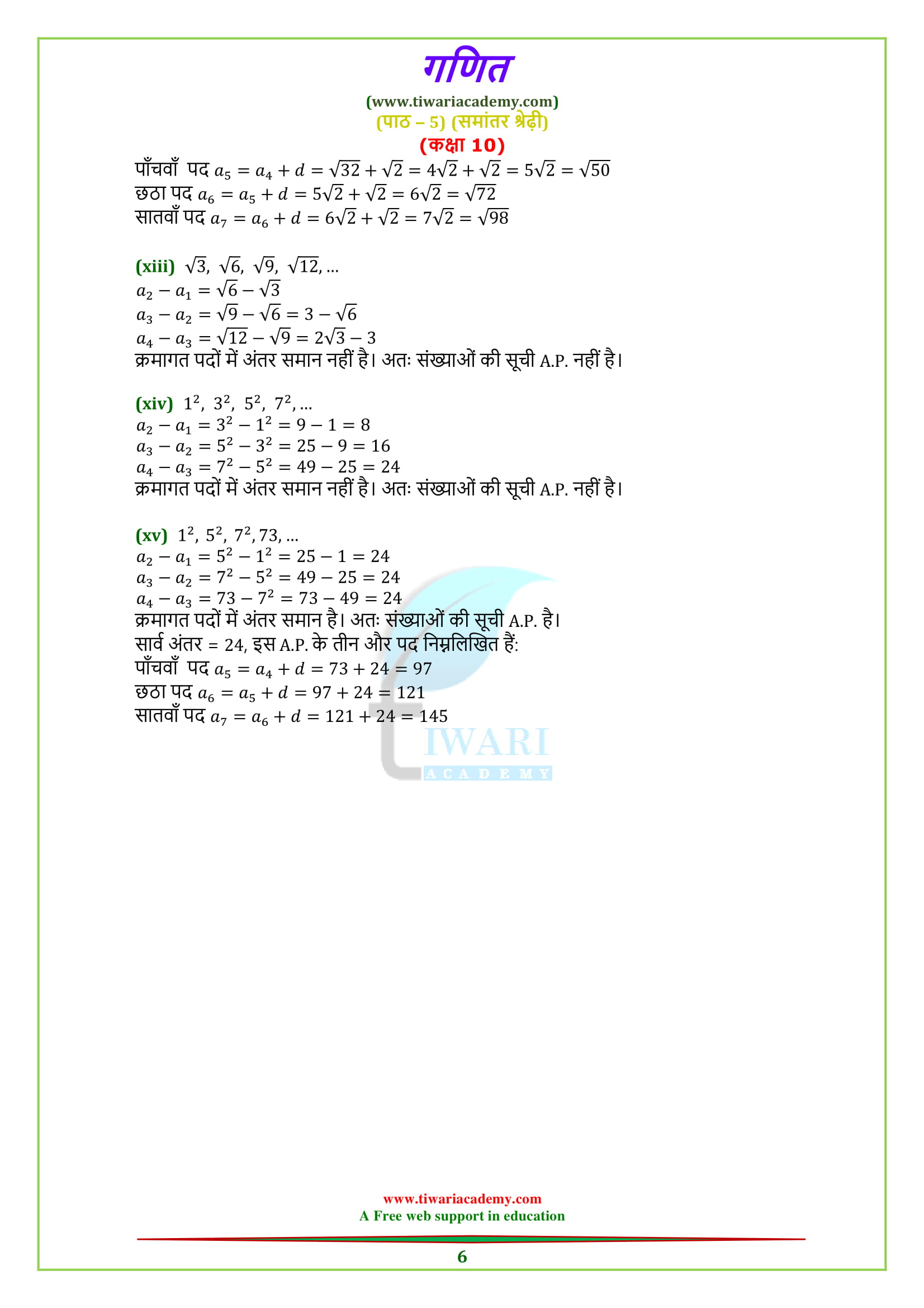NCERT Solutions for class 10 Maths Chapter 5 Exercise 5.1 Arithmetic Progressions in Hindi Medium and English Medium for session 2022-2023.

• ## NCERT Solutions for class 10 Maths Chapter 5 Exercise 5.1

### 10 Maths Chapter 5 Exercise 5.1 Solutions

NCERT Solutions for class 10 Maths Chapter 5 Exercise 5.1 Arithmetic Progressions in English as well as Hindi medium free to download or View in Video Format or digital contents for online use. Move Class 10 Maths Chapter 5 main page for other exercises whether download or online study. Visit to Discussion Forum to discuss your questions and reply to the questions asked by others. NCERT Solutions are applicable for CBSE, MP Board and UP Board students who are using CBSE NCERT Books for 2022-2023.

#### Important Questions on AP

1. Find 5th term of an A.P. whose nth term is 3n – 5. [Answer: 10]
2. Find the sum of first 10 even numbers. [Answer: 110]
3. Write the nth term of odd numbers. [Answer: 2n – 1]
4. Write the sum of first n natural numbers. [Answer: n(n + 1)/2]
5. Write the sum of first n even numbers. [Answer: n(n + 1)]
6. Find the nth term of the A.P.–10, – 15, –20, – 25… [Answer: – 5(n + 1)]
7. Write the common difference of an A.P. whose nth term is an = 3n + 7. [Answer: 3]
8. What will be the value of a8 – a4 for the following A.P. 4, 9, 14… 254. [Answer: 20]
10. Find the 20th term from the last term of the A.P. 3,8,13… 253. [Answer: 158]
##### Questions from Board Papers

1. Which term of the A.P. 5, 15, 25 …will be 130 more than its 31st term? [Answer: 44th]
2. The first term, common difference and last term of an A.P. are 12, 6 and 252 respectively, Find the sum of all terms of this A.P. [Answer: 5412]
3. Find the sum of first 15 multiples of 8. [Answer: 540]
4. Is the sequence formed in the following situations an AP.
(i) Number of students left in the school auditorium from the total strength of 1000 students when they leave the auditorium in batches of 25.
(ii) The amount of money in the account every year when ₹100 are deposited annually to accumulate at compound interest at 4% per annum. [Answer: (i) Yes, (ii) No]
5. Find the sum of even positive integers between 1 and 200. [Answer: 9900]
6. If 4m + 8, 2m² + 3m + 6, 3m² + 4m + 4 are three consecutive terms of an A.P. find m. [Answer: m = 0, 2]

Download NCERT Books and Offline Apps 2022-23 based on new CBSE Syllabus. Ask your doubts related to NIOS or CBSE Board and share your knowledge with your friends and other users through Discussion Forum.

### What do students learn from exercise 5.1 of class 10th Maths?

After completing exercise 5.1 of class 10th math, students can form Arithmetic Progression if first term and common difference is given. Students can find first term and common difference if Arithmetic Progression is given. Also student can tell whether the given list of numbers is Arithmetic Progression or not.

### How many examples and questions are there in exercise 5.1 of class 10th math?

There are two examples (Examples 1 and 2) and four questions in exercise 5.1 of class 10th math. Both the examples are easy to understand as well as good for practice.

### Which is the most favourite questions of exercise 5.1 of 10 Maths?

Question 2, 3 and 4 of exercise 5.1 (class 10 math) are student’s favourite questions because these questions are very easy and students solve these questions without any difficulty.

### Is exercise 5.1 of class 10 math easy?

Exercise 5.1 of class 10 math is the easiest exercise. But difficulty level of anything varies from student to student. So, Exercise 5.1 (chapter 5) of class 10th mathematics is easy or not depends on students also.